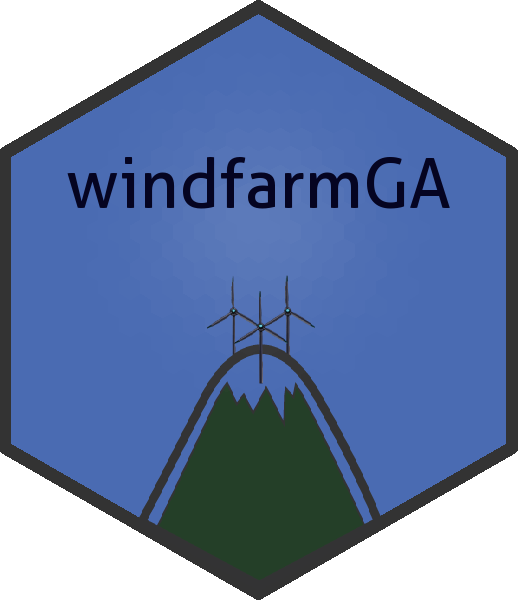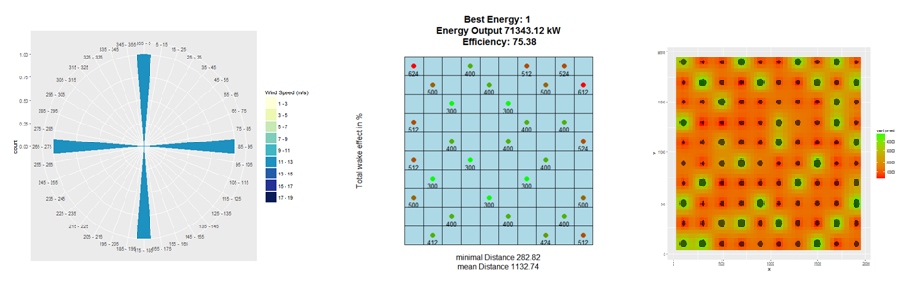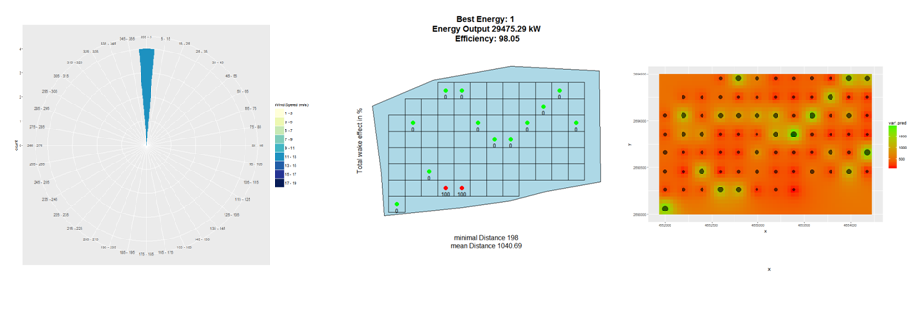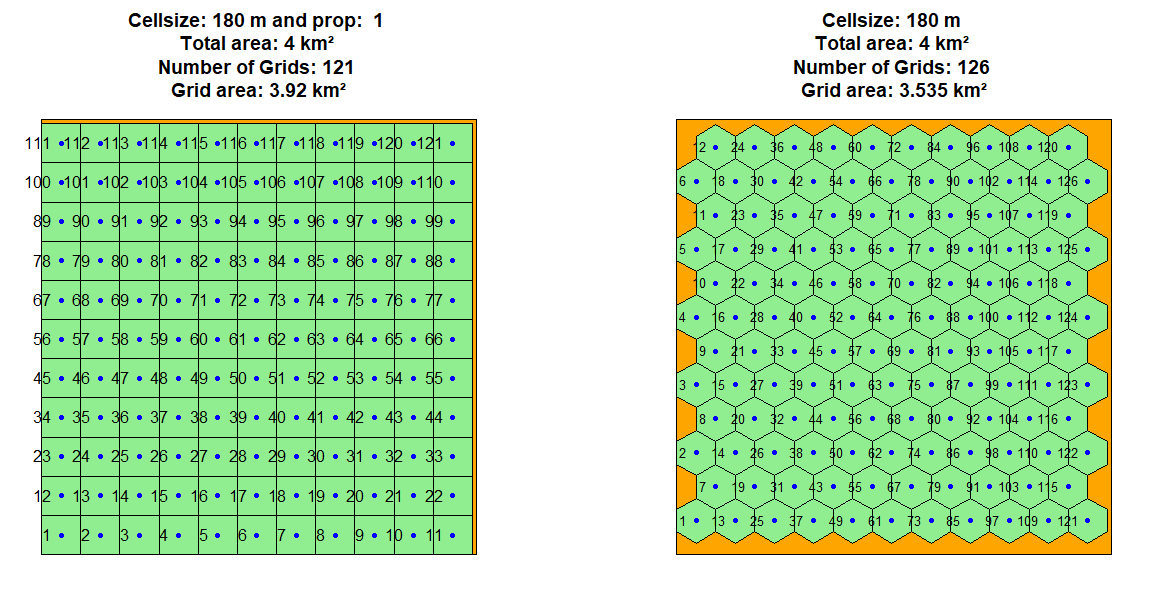# Windfarmga

R Package to Optimize Windfarm Layouts
Alternatives To Windfarmga
Gridcal358
a day ago277December 01, 202352lgpl-3.0Python
GridCal, a cross-platform power systems software written in Python with user interface, used in academia and industry.
Sgpp55
2 years ago2March 27, 202036otherC++
SG⁺⁺ – the numerical library for Sparse Grids in all their variants.
Edisgo32
16 months ago14July 27, 202153agpl-3.0Python
Optimization of flexibility options and grid expansion for distribution grids based on PyPSA
Etrago26
110 hours ago9November 21, 202384agpl-3.0Python
Optimization of flexibility options for transmission grids based on PyPSA
Microgrids25
6 years agoeupl-1.1Python
Library of tools for the simulation and optimization of microgrids
Windfarmga24
7 months ago7August 27, 20194otherR
R Package to Optimize Windfarm Layouts
Fluidml19
2 months ago10October 20, 20231apache-2.0Python
FluidML is a lightweight framework for developing machine learning pipelines.
Ego16
16 months ago4December 10, 20189agpl-3.0Python
A cross-grid-level electricity grid and storage optimization tool
Gameoflife15
4 years ago3November 18, 2015mitPython
Conway's Game of Life
Nips2014 Survey12
8 years ago
Survey of hyperparameter optimization use in NIPS2014
Alternatives To Windfarmga
Select To Compare

Alternative Project Comparisons

# windfarmGAGenetic algorithm to optimize the layout of windfarms. The package is hosted on CRAN

# Installation

``````devtools::install_github("YsoSirius/windfarmGA")
``````

and the CRAN-version with:

``````install.packages("windfarmGA")
``````

# Description

The genetic algorithm is designed to optimize wind farms of any shape. It requires a predefined number of turbines, a uniform rotor radius and an average wind speed per wind direction. It can include a terrain effect model, which downloads an 'SRTM' elevation model and a 'Corine Land Cover' raster automatically. The elevation model is used to find mountains and valleys and to adjust the wind speeds accordingly by 'wind multipliers' and to determine the air densities at rotor heights. The land cover raster with an additional elevation roughness value is used to re-evaluate the surface roughness and to individually determine the wake-decay constant for each turbine.

To start an optimization, either the function `windfarmGA` or `genetic_algorithm` can be used. The function `windfarmGA` checks the user inputs interactively and then runs the function `genetic_algorithm`. If the input parameters are already known, an optimization can be run directly via `genetic_algorithm`.Since version 1.1, hexagonal grid cells are possible, with their center points being possible locations for wind turbines. Furthermore, rasters can be included, which contain information on the Weibull parameters. For Austria this data is already included in the package.

## Create an input Polygon

• Input Polygon by source
``````library(sf)
dsn <- "Path to the Shapefile"
layer <- "Name of the Shapefile"
Polygon1 <- sf::st_read(dsn = dsn, layer = layer)
plot(Polygon1, col = "blue")
``````
• Or create a random Polygon
``````library(sf)
Polygon1 <- sf::st_as_sf(sf::st_sfc(
sf::st_polygon(list(cbind(
c(0, 0, 2000, 2000, 0),
c(0, 2000, 2000, 0, 0)))),
crs = 3035
))
plot(Polygon1, col = "blue", axes = TRUE)
``````

## Create random Wind data

• Exemplary input Wind data with uniform wind speed and single wind direction
``````wind_df <- data.frame(ws = c(12, 12), wd = c(0, 0), probab = c(25, 25))
windrosePlot <- plot_windrose(data = wind_df, spd = wind_df\$ws,
dir = wind_df\$wd, dirres=10, spdmax = 20)
``````
• Exemplary input Wind data with random wind speeds and random wind directions
``````wind_df <- data.frame(ws = sample(1:25, 10), wd = sample(1:260, 10)))
windrosePlot <- plot_windrose(data = wind_df, spd = wind_df\$ws,
dir = wind_df\$wd)
``````

## Grid Spacing

### Rectangular Grid Cells

Verify that the grid spacing is appropriate. Adapt the following input variables if necessary:

• Rotor: The rotor radius in meters.
• fcrR: The grid spacing factor, which should at least be 2, so that a single grid covers at least the whole rotor diameter.
• prop: The proportionality factor used for grid calculation. It determines the minimum percentage that a grid cell must cover of the area.

Make sure that the Polygon is projected in meters.

``````Rotor <- 20
fcrR <- 9
Grid <- grid_area(Polygon1, size = (Rotor * fcrR), prop = 1, plotGrid = TRUE)
str(Grid)
``````

### Hexagonal Grid Cells

``````Rotor <- 20
fcrR <- 9
HexGrid <- hexa_area(Polygon1, size = (Rotor * fcrR), plotGrid = TRUE)
str(HexGrid)
``````## Terrain Effect Model

If the input variable topograp for the functions `windfarmGA` or `genetic_algorithm` is TRUE, the genetic algorithm will take terrain effects into account. For this purpose an elevation model and a Corine Land Cover raster are downloaded automatically, but can also be given manually. ( Download a CLC raster ).

If you want to include your own Land Cover Raster, you must assign the Raster Image path to the input variable sourceCCL. The algorithm uses an adapted version of the Raster legend ("clc_legend.csv"), which is stored in the package subdirectory (/extdata). To use own values for the land cover roughness lengths, insert a column named Rauhigkeit_z to the .csv file. Assign a surface roughness length to all land cover types. Be sure that all rows are filled with numeric values and save the .csv file with ";" delimiter. Assign the .csv file path to the input variable sourceCCLRoughness.

## Start an Optimization

An optimization run can be initiated with the following functions:

• genetic_algorithm
• windfarmGA

### Function calls for windfarmGA

• without terrain effects
``````result <- windfarmGA(Polygon1 = Polygon1, n = 12, Rotor = 20, fcrR = 9, iteration = 10,
vdirspe = wind_df, crossPart1 = "EQU", selstate = "FIX", mutr = 0.8,
Proportionality = 1, SurfaceRoughness = 0.3, topograp = FALSE,
elitism =TRUE, nelit = 7, trimForce = TRUE,
referenceHeight = 50, RotorHeight = 100)
``````
• with terrain effects
``````sourceCCL <- "Source of the CCL raster (TIF)"
sourceCCLRoughness <- "Source of the Adaped CCL legend (CSV)"

result <- windfarmGA(Polygon1 = Polygon1, n = 12, Rotor = 20, fcrR = 9, iteration = 10,
vdirspe = wind_df, crossPart1 = "EQU", selstate = "FIX", mutr = 0.8,
Proportionality = 1, SurfaceRoughness = 0.3, topograp = TRUE,
elitism = TRUE, nelit = 7, trimForce = TRUE,
referenceHeight = 50, RotorHeight = 100, sourceCCL = sourceCCL,
sourceCCLRoughness = sourceCCLRoughness)
``````

### Function calls for genetic_algorithm

• without terrain effects
``````result <- genetic_algorithm(Polygon1 = Polygon1, n = 12, Rotor = 20, fcrR = 9, iteration = 10,
vdirspe = wind_df, crossPart1 = "EQU", selstate = "FIX", mutr =0.8,
Proportionality = 1, SurfaceRoughness = 0.3, topograp = FALSE,
elitism = TRUE, nelit = 7, trimForce = TRUE,
referenceHeight = 50, RotorHeight = 100)
``````
• with terrain effects
``````sourceCCL <- "Source of the CCL raster (TIF)"
sourceCCLRoughness <- "Source of the Adaped CCL legend (CSV)"
result <- genetic_algorithm(Polygon1 = Polygon1, n= 12, Rotor = 20, fcrR = 9, iteration = 10,
vdirspe = wind_df, crossPart1 = "EQU", selstate = "FIX", mutr = 0.8,
Proportionality = 1, SurfaceRoughness = 0.3, topograp = TRUE,
elitism = TRUE, nelit = 7, trimForce = TRUE,
referenceHeight = 50, RotorHeight = 100, sourceCCL = sourceCCL,
sourceCCLRoughness = sourceCCLRoughness)
``````
``````## Run an optimization with your own Weibull parameter rasters. The shape and scale
## parameter rasters of the weibull distributions must be added to a list, with the first
## list item being the shape parameter (k) and the second list item being the scale
## parameter (a). Adapt the paths to your raster data and run an optimization.
kraster <- "/..pathto../k_param_raster.tif"
araster <- "/..pathto../a_param_raster.tif"
weibullrasters <- list(raster(kraster), raster(araster))

result_weibull <- genetic_algorithm(Polygon1 = Polygon1, GridMethod ="h", n=12,
fcrR=5, iteration=10, vdirspe = wind_df, crossPart1 = "EQU",
selstate="FIX", mutr=0.8, Proportionality = 1, Rotor=30,
SurfaceRoughness = 0.3, topograp = FALSE,
elitism=TRUE, nelit = 7, trimForce = TRUE,
referenceHeight = 50,RotorHeight = 100,
weibull = TRUE, weibullsrc = weibullrasters)
plot_windfarmGA(result = result_weibull, Polygon1 = Polygon1)
``````

The argument GridMethod, weibull, weibullsrc can also be given to the function `windfarmGA`.

#### Plot the Results on a Leaflet Map

``````## Plot the best wind farm on a leaflet map (ordered by energy values)
plot_leaflet(result = resulthex, Polygon1, which = 1)

## Plot the last wind farm (ordered by chronology).
plot_leaflet(result = resulthex, Polygon1, orderitems = FALSE, which = 1)
``````

## Plotting Methods of the Genetic Algorithm

Several plotting functions are available:

`````` - plot_windfarmGA(result, Polygon1)
- plot_result(result, Polygon1, best = 1)
- plot_evolution(result, ask = TRUE, spar = 0.1)
- plot_development(result)
- plot_parkfitness(result, spar = 0.1)
- plot_fitness_evolution(result)
- plot_cloud(result, pl = TRUE)
- plot_heatmap(result = result, si = 5)
- plot_leaflet(result = result, Polygon1 = Polygon1, which = 1)
``````

A full documentation of the genetic algorithm is given in my master thesis.

# Shiny Windfarm Optimization

I also made a Shiny App for the Genetic Algorithm. Unfortunately, as an optimization takes quite some time and the app is currently hosted by shinyapps.io under a public license, there is only 1 R-worker at hand. So only 1 optimization can be run at a time.

# Full Optimization example:

``````library(sf)
library(windfarmGA)

Polygon1 <- sf::st_as_sf(sf::st_sfc(
sf::st_polygon(list(cbind(
c(4651704, 4651704, 4654475, 4654475, 4651704),
c(2692925, 2694746, 2694746, 2692925, 2692925)))),
crs = 3035
))
plot(Polygon1, col = "blue", axes = TRUE)

wind_df <- data.frame(ws = 12, wd = 0)
windrosePlot <- plot_windrose(data = wind_df, spd = wind_df\$ws,
dir = wind_df\$wd, dirres = 10, spdmax = 20)
Rotor <- 20
fcrR <- 9
Grid <- grid_area(shape = Polygon1, size = (Rotor*fcrR), prop = 1, plotGrid = TRUE)

result <- genetic_algorithm(Polygon1 = sp_polygon,
n = 20,
Rotor = Rotor, fcrR = fcrR,
iteration = 50,
vdirspe = wind_df,
referenceHeight = 50, RotorHeight = 100)

# The following function will execute all plotting function further below:
plot_windfarmGA(result, Polygon1, whichPl = "all", best = 1, plotEn = 1)

# The plotting functions can also be called individually:
plot_result(result, Polygon1, best = 1, plotEn = 1, topographie = FALSE)
plot_evolution(result, ask = TRUE, spar = 0.1)
plot_parkfitness(result, spar = 0.1)
plot_fitness_evolution(result)
plot_cloud(result, pl = TRUE)
plot_heatmap(result = result, si = 5)
plot_leaflet(result = result, Polygon1 = Polygon1, which = 1)
``````
Popular Grid Projects
Popular Optimization Projects
Popular User Interface Components Categories
Related Searches

Get A Weekly Email With Trending Projects For These Categories
No Spam. Unsubscribe easily at any time.
R
Grid
Optimization
Genetic Algorithm
Terrain
Raster